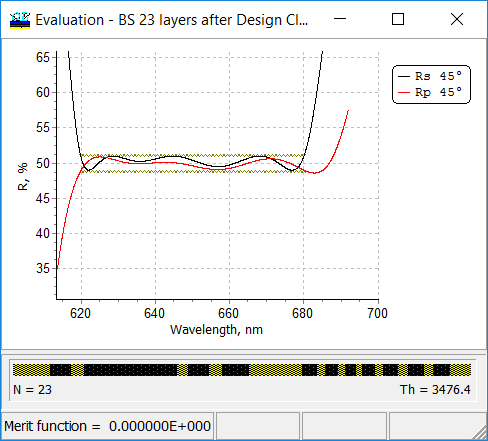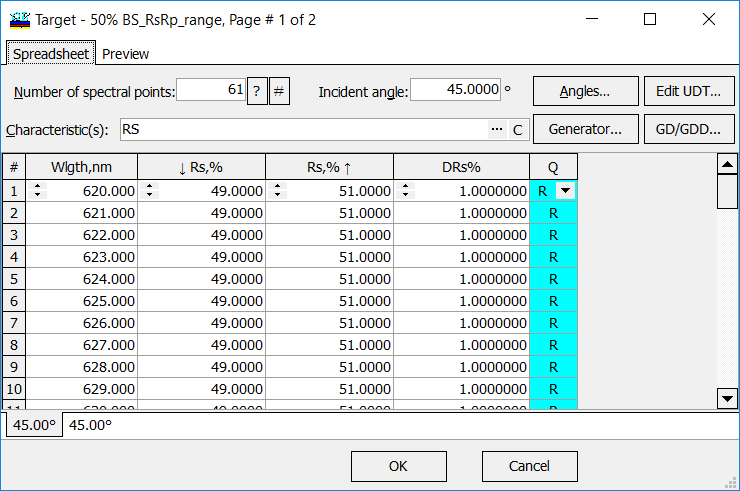### Range TargetsOptiLayer allows you to specify targets for cases when your spectral characteristic $$S(\lambda)$$ should satisfy the following conditions: $S(\lambda_j)\ge a_j,$ $S(\lambda_j)\le b_j,$ $a_j\le S(\lambda_j)\le b_j$ Example (left panel). Beamplitter design satisfies range target conditions: $49\%\le R(\lambda_j)\le 51\%, \lambda_j=620+(j-1)$ Design targets (and therefore a merit function) can be modified using qualifiers A, B or R. A corresponds to the Bypass Above option. It means that OptiLayer bypasses the target when the corresponding characteristic is greater than a target value. B corresponds to the Bypass Below option. It means that OptiLayer bypasses the target when the corresponding characteristic is less than a target value. R corresponds to the Range option. It means that OptiLayer bypasses the target when the corresponding characteristic belongs to a given segment (see right panel). An empty qualifier (blank) corresponds to a conventional target.